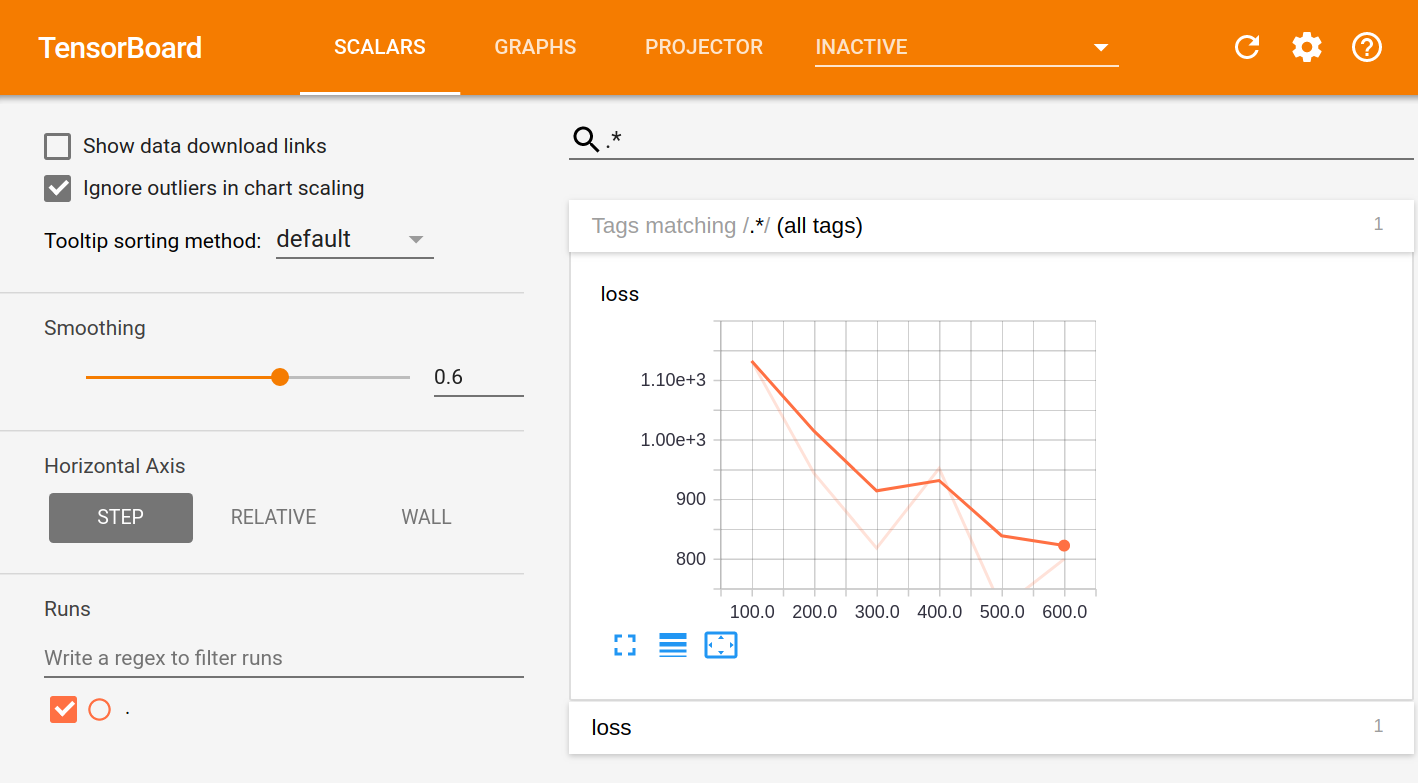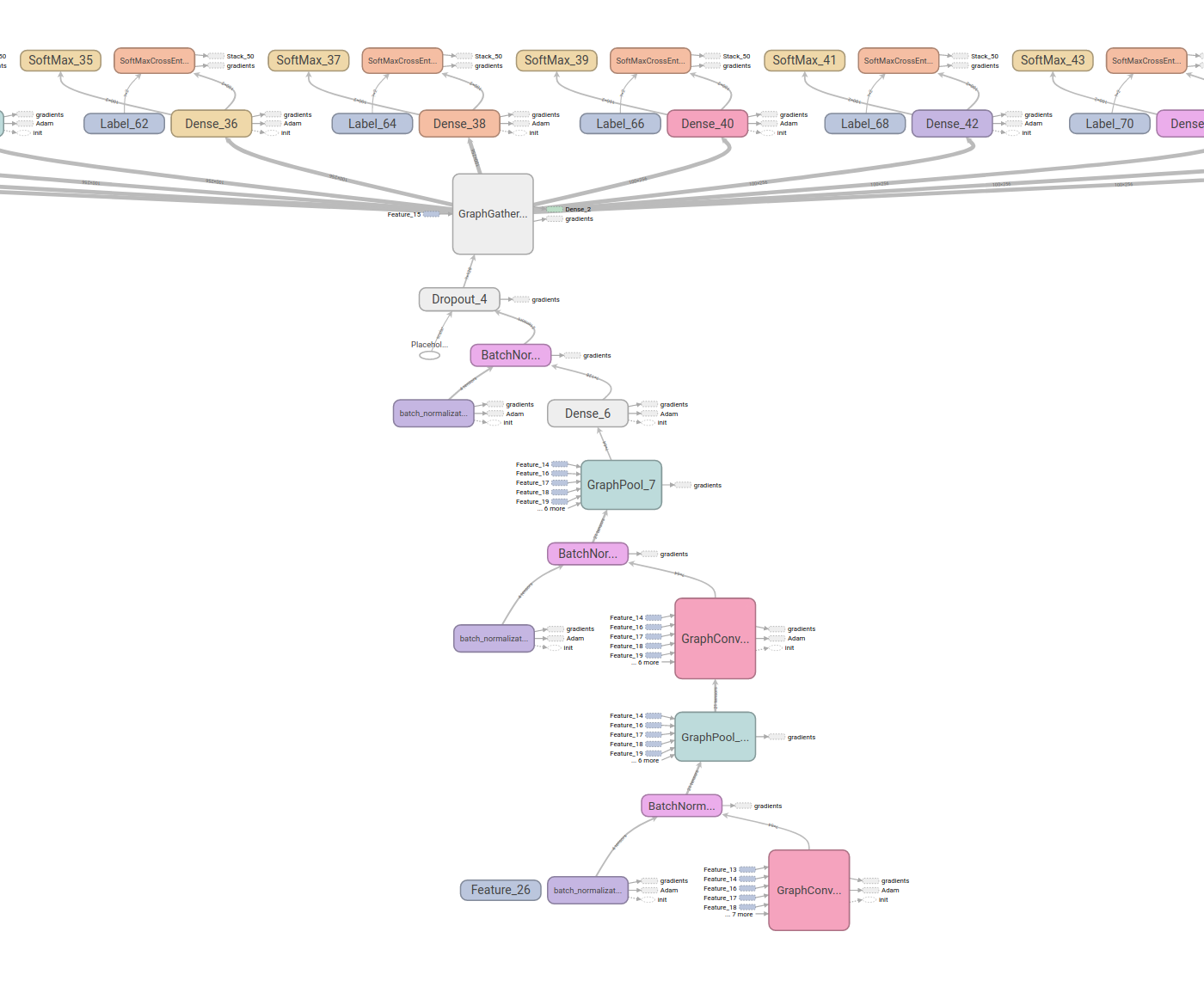# Using Tensorboard in DeepChem

DeepChem Neural Networks models are built on top of tensorflow. Tensorboard is a powerful visualization tool in tensorflow for viewing your model architecture and performance.

In this tutorial we will show how to turn on tensorboard logging for our models, and go show the network architecture for some of our more popular models.

The first thing we have to do is load a dataset that we will monitor model performance over.

``````import deepchem as dc
from deepchem.models.tensorgraph.models.graph_models import GraphConvTensorGraph

train_dataset, valid_dataset, test_dataset = tox21_datasets

``````
``````Loading dataset from disk.
``````

Now we will create our model with tensorboard on. All we have to do to turn tensorboard on is pass the tensorboard=True flag to the constructor of our model

``````# Construct the model with tensorbaord on

# Fit the model
model.fit(train_dataset, nb_epoch=10)
``````
``````Starting epoch 0
Starting epoch 1
Starting epoch 2
Starting epoch 3
Starting epoch 4
Starting epoch 5
Starting epoch 6
Starting epoch 7
Starting epoch 8
Starting epoch 9
Ending global_step 630: Average loss 940.645
TIMING: model fitting took 52.005 s

940.6450127495659
``````

### Viewing the Tensorboard output

When tensorboard is turned on we log all the files needed for tensorboard in model.model_dir. To launch the tensorboard webserver we have to call in a terminal

``````tensorboard --logdir=model.model_dir
``````